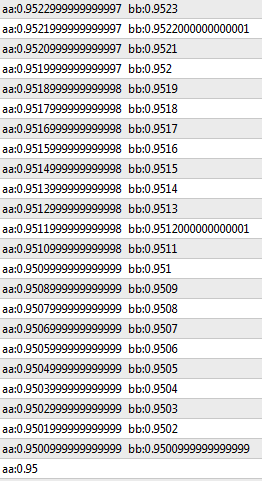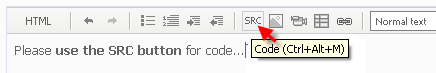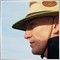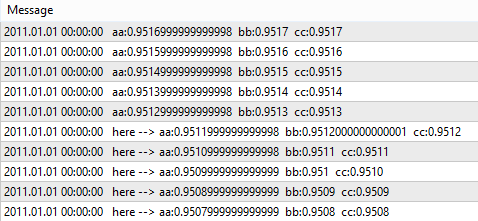# Bugs in MQL 5 for double value and NormalizeDouble function147

Hi,

The following is a simple program to print the double value after normalized, it should give ONLY 4 decimal points but it is not.  (see result image)

Instead of the value 0.9512, the value becomes 0.95120000000001, it will cause the program bugs if I use bb for if statement.  Pls advise if that is the bugs in MQL 5.  The same program has no problem at all in MQL 4.

```double bb;
double aa = 0.9500;
Print("aa:",aa);
for (int x=0; x<100; x++) {
aa = aa + 0.0001;
bb = NormalizeDouble(aa,4);
if (bb <= 0.9512) { /* other programs */}
Print("aa:",aa,"  bb:",bb);

}```

Regards,

Toyogo40731

Please use SRC button when you are posting code.Why do you think it should only print 4 decimals ?147

angevoyageur:

Please use SRC button when you are posting code.

Why do you think it should only print 4 decimals ?

Hi,

I use the NormalizeDouble function to change the bb's accuracy to 4 decimal points.  You can see from the image result that most of the bb are 4 decimal points except few are not.  Indeed, the printing of the bb of more than 4 decimal is not what I concern.  I am more concern that the value of bb has been changed from 0.9512 to 0.95120000000001.  This has caused the program bugs if I use bb for if statement.

Regards,

ToyogoDocumentation on MQL5: Conversion Functions / NormalizeDouble
• www.mql5.com
Conversion Functions / NormalizeDouble - Documentation on MQL540731

toyogo00:

Hi,

I use the NormalizeDouble function to change the bb's accuracy to 4 decimal points.  You can see from the image result that most of the bb are 4 decimal points except few are not.  Indeed, the printing of the bb of more than 4 decimal is not what I concern.  I am more concern that the value of bb has been changed from 0.9512 to 0.95120000000001.  This has caused the program bugs if I use bb for if statement.

Regards,

Toyogo

This is definitively not a bug. Read the documentation of Print().

Data of double type are shown with the accuracy of up to  16 digits after a decimal point.

What is true for one language isn't necessarily true for another, in MQL4 Print only output double with 4 decimals.

You have also to be aware of what is a double.17296

toyogo00:

Hi,

I use the NormalizeDouble function to change the bb's accuracy to 4 decimal points.  You can see from the image result that most of the bb are 4 decimal points except few are not.  Indeed, the printing of the bb of more than 4 decimal is not what I concern.  I am more concern that the value of bb has been changed from 0.9512 to 0.95120000000001.  This has caused the program bugs if I use bb for if statement.

This is the normal behaviour of doubles,  not just in mql5 or mql5 . . .  you should read this  Can price != price ?  and this   floating point numbers  and this thread  https://www.mql5.com/en/forum/11387/page9
Can price != price ? - MQL4 forum
• www.mql5.com
Can price != price ? - MQL4 forum147

RaptorUK:
This is the normal behaviour of doubles,  not just in mql5 or mql5 . . .  you should read this  Can price != price ?  and this   floating point numbers  and this thread  https://www.mql5.com/en/forum/11387/page9

Hi,

If this is a normal bevaviour of doubles, what is a work-around?  I assign a variable aa = 0.9520 + 0.0001 but it stored as aa=0.952099999999998, I applied bb = NormalizeDouble(aa), it stored as bb=0.95210000000000001.  How to make bb = 0.9521?

Regards.

Toyogo29826

```double bb;
double aa = 0.9500;
Print("aa:",aa);
for (int x=0; x<100; x++) {
aa = aa + 0.0001;
bb = NormalizeDouble(aa,4);
if (bb <= 0.9512) { Print("here --> aa:",aa,"  bb:",bb,"  cc:",DoubleToString(bb,4)); }
else { Print("aa:",aa,"  bb:",bb,"  cc:",DoubleToString(bb,4)); }
}```Nothing wrong with NormalizeDouble - it is "Print".17296

toyogo00:

Hi,

If this is a normal bevaviour of doubles, what is a work-around?  I assign a variable aa = 0.9520 + 0.0001 but it stored as aa=0.952099999999998, I applied bb = NormalizeDouble(aa), it stored as bb=0.95210000000000001.  How to make bb = 0.9521?

You cannot,  read the links I gave you, it's a result of how double values are stored.